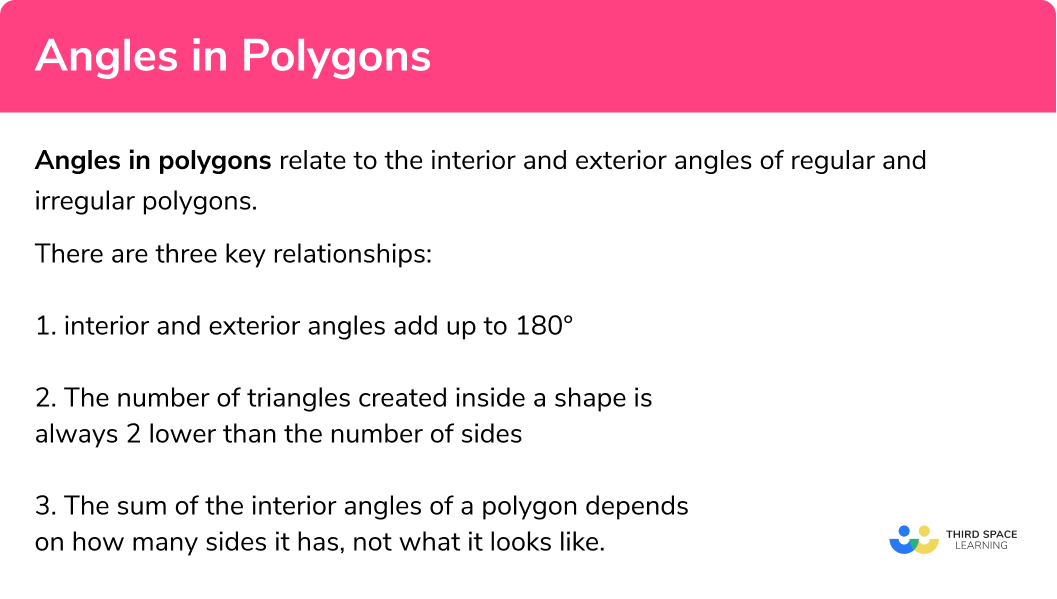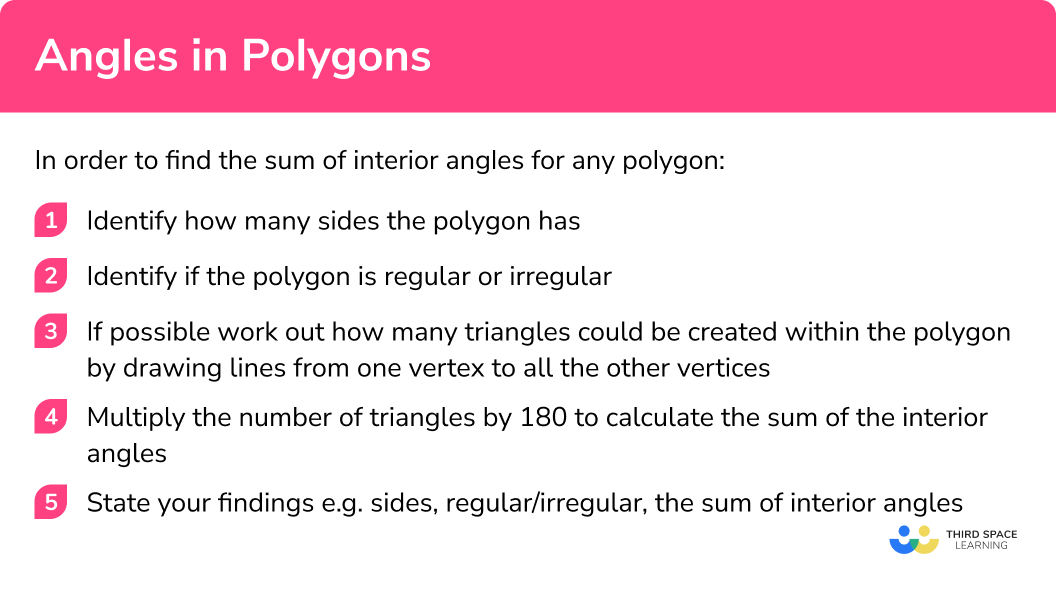# Angles In Polygons

Here we will learn about angles in polygons including how to calculate angles in polygons using a variety of methods and an overview of interior and exterior angles

There are also angles in polygons worksheets based on Edexcel, AQA and OCR exam questions, along with further guidance on where to go next if you’re still stuck.

## What are angles in polygons?

Angles in polygons relate to the interior and exterior angles of regular and irregular polygons.

Interior angles are the angles within a polygon made by two sides.
We can calculate the sum of the interior angles of a polygon by subtracting 2 from the number of sides and then multiplying by 180º.

Step-by-step guide: Interior angles of a polygon

Exterior angles are the angles between a polygon and the extended line from the next side.
The sum of the exterior angles of a polygon is always equal to 360º.

Step-by-step guide: Exterior angles of a polygon

A polygon is a two dimensional shape with at least three sides, where the sides are all straight lines.
‘Poly’ comes from the greek for ‘many’ whilst ‘gon’ means ‘angles’.
You will be familiar with many types of polygons such as triangle, rectangle and pentagon.

Regular polygons have all angles are that are equal in size and all sides that are equal in length.

Irregular polygons have angles that are not equal in size and sides that are not equal in length.

### What are angles in polygons## Sum of the interior angles of a polygon

The ‘sum of interior angles’ of a polygon means finding the total of all the angles in a polygon. This is the key step in helping us solve many problems involving angles in polygons.

We know that the sum of all the angles in a triangle is equal to 180º, but what about angles in a quadrilateral? A regular pentagon? Or even an irregular octagon?

Sum of the angles in a triangle:

We know that the three angles in any triangle add up to 180º.

Mathematically we would say:

“The sum of interior angles for a triangle is 180 degrees”.

Step-by-step guide: Angles in a triangle

Sum of the angles in a quadrilateral:

A quadrilateral is a four sided shape. We can ‘split’ a quadrilateral into two triangles by drawing a line from one corner to an opposite one.

If the sum of interior angles one triangle is 180º , then the sum of the interior angles of two triangles is 180º × 2 = 360º.

So the sum of the interior angles of quadrilateral is 360º.

Step-by-step guide: Angles in a quadrilateral

Using our knowledge of triangles we can find the sum of the interior angles of any polygon by splitting it into triangles.

Step-by-step guide: Angles in a pentagon

Step-by-step guide: Angles in a hexagon

### Key relationships

Interior and exterior angles lie on a straight line. This means that when added together they will equal 180º.

This is useful when working on more complex questions.

Here is a list of all the polygons we will work with in this lesson:

Notice how the number of triangles created is always 2 lower than the number of sides.

Despite these two decagons (10 sided shapes) looking very different, the sum of their interior angles is the same.

## How to find the sum of the interior angles of a polygon

In order to find the sum of interior angles for any polygon you should:

1. Identify how many sides the polygon has.
2. Identify if the polygon is regular or irregular.
3. If possible work out how many triangles could be created within the polygon by drawing lines from one vertex to all the other vertices.
4. Multiply the number of triangles by 180 to calculate the sum of the interior angles.
5. State your findings e.g. sides, regular/irregular, the sum of interior angles.

### How to find the sum of the interior angles for a polygon## Angles in polygons examples

Find the sum of interior angles for this polygon.

1. Identify how many sides the polygon has.

This polygon has four sides.

2 Identify if the polygon is regular or irregular.

This polygon is irregular as all the sides are not of equal length.

3 If possible work out how many triangles could be created within the polygon by drawing lines from one corner to all the other vertices.

The polygon can be broken up into two triangles.

4 Multiply the number of triangles by 180 to get the sum of the interior angles.

$180^{\circ} × 2 = 360^{\circ}$

5 State your findings e.g. sides, regular/irregular, the sum of interior angles.

The polygon is a irregular quadrilateral (specifically called a parallelogram as both opposite sides are parallel) with a sum of interior angles of 360º.

### Example 2: regular decagon

This polygon has 10 sides.

This polygon is regular as all the sides are of equal length and the angles are of equal size.

The polygon can be broken up into eight triangles.

$180^{\circ} × 8 = 1440^{\circ}$

The polygon is a regular 10 sided polygon (decagon) with a sum of interior angles of 1440º.

### Example 3: irregular pentagon with concave angle

This polygon has five sides.

This polygon is irregular as all the sides are not of equal length and the angles are not of equal size

The polygon can be broken up into three triangles.

$180^{\circ} × 3 = 540^{\circ}$

The polygon is a irregular five sided polygon (pentagon) with a sum of interior angles of 540º.

### Example 4: complex polygon

This polygon has 10 sides

This polygon is irregular as all the angles are not of equal size.

The polygon can be broken up into eight triangles.

Notice how we had to do this differently to previous examples. But we have not created any new angles because our lines do not cross.

$180^{\circ} × 8 = 1440^{\circ}$

The polygon is a irregular 10 sided polygon with a sum of interior angles of 1440º.

STOP AND THINK: Notice how this is the same sum of interior angles for example 2. This is because the polygon has the same number of sides.

### Example 5: finding an exterior angle

The sum of interior angle in a triangle is 180º.

An equilateral triangle has 3 angles of equal size.

Therefore each interior angle is 60º because 180 ÷ 3 = 60.

Therefore one exterior angle is equal to 180 – 60 because the interior angle and exterior angle of a polygon lie on a straight line.

Therefore one exterior angle is 120º.

### Example 6: finding the sum of interior angles of larger polygons

To work out the number of triangles a polygon can be split into we subtract 2.

A 30 sided polygon can be split into 30 − 2 = 28 triangles.

Therefore the sum of its interior angles can be found by 28 × 180º = 5040º.

The sum of interior angles of a 30 sided polygon is 5040º.

This will be looked at in more detail in the lesson on interior angles in a polygon.

### Example 7: finding the sum of interior angles of a complex polygon

This polygon has 6 sides. The sides are not all the same length so this polygon is an irregular hexagon.

To work out the number of triangles a polygon can be split into we subtract 2 from the number of sides it has.

A 6 sided polygon can be split into 6 − 2 = 4 triangles.

Therefore the sum of its interior angles can be found by 4 x 180º = 720º.

The sum of interior angles in a hexagon is 720º.

### Common misconceptions

• Miscounting the number of sides

• Misidentifying if a polygon is regular or irregular

• Incorrectly assuming all the angles are the same size

• Crossing lines when drawing the triangles, this creates false interior angles

### Practice angles in polygons questions

1. Is a square a regular or irregular polygon?

RegularIrregularAll the side lengths are the same, and all angles are right angles, hence a square is a regular polygon.

2. Is a semi circle a polygon?

YesNoA semicircle has a side which is not straight, so it is not a polygon.

3. What is the sum of interior angles for a triangle?

90^{\circ}180^{\circ}360^{\circ}270^{\circ}The angles in a triangle add up to 180^{\circ}.

4. What is the sum of interior angles for a regular hexagon?

720^{\circ}540^{\circ}360^{\circ}1080^{\circ}We know the angles in a triangle add up to 180^{\circ} . A regular hexagon can be divided into 4 triangles, and four lots of 180 is 720 .

5. What is the sum of interior angles for an irregular hexagon?

720^{\circ}540^{\circ}360^{\circ}1080^{\circ}We know the angles in a triangle add up to 180^{\circ} . An irregular hexagon can be divided into 4 triangles, and four lots of 180 is 720 .

6. What is the sum of interior angles for a regular 12 sided polygon?

2700^{\circ}2160^{\circ}1800^{\circ}1080^{\circ}We know the angles in a triangle add up to 180^{\circ} . A 12 sided polygon can be divided into 10 triangles, and ten lots of 180 is 1800 .

7. What is the sum of interior angles for a regular 25 sided polygon?

4500^{\circ}4140^{\circ}9000^{\circ}3600^{\circ}We know the angles in a triangle add up to 180^{\circ} . A 25 sided polygon can be divided into 23 triangles, and 23 lots of 180 is 4140 .

### Angles in polygons GCSE questions

1.  Each exterior angle of a regular polygon is 15^{\circ} .
Work out the number of sides the polygon has.

(2 marks)

360 \div 15

(1)

24

(1)

2.  Each of the interior angles of a regular polygon is 140^{\circ} . Show that this polygon has 9 sides

(2 marks)

Exterior angle = 40 seen or implied

(1)

360 \div 40=9

(1)

3.  In a regular polygon each exterior angle is 18^{\circ} . Find the sum of interior angles for this polygon

(3 marks)

360 \div 18 \quad \text { or implied by "20" }

(1)

180 \times 18

(1)

3240

(1)

## Learning checklist

You have now learned how to:

• Use conventional terms for geometry e.g. regular or irregular
• Derive and apply the properties and definitions of: special types of quadrilaterals
• Knowing names and properties of polygons
• Calculate the sum of interior angles for a regular polygon
• Calculate the sum of interior angles for a irregular polygon

## Still stuck?

Prepare your KS4 students for maths GCSEs success with Third Space Learning. Weekly online one to one GCSE maths revision lessons delivered by expert maths tutors.

Find out more about our GCSE maths tuition programme.